# Difference between revisions of "Normal matrices"

A complex square matrix A is a normal matrix if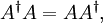$A^\dagger A=AA^\dagger ,$

where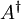$A^\dagger$ is the conjugate transpose of A. That is, a matrix is normal if it commutes with its conjugate transpose: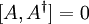$[A,A^\dagger]=0$.

Normal matrices are precisely those to which the spectral theorem applies: a matrix$A$ is normal if and only if it can be represented by a diagonal matrix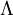$\Lambda$ and a unitary matrix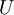$U$ by the formula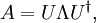$A = U \Lambda U^\dagger,$

where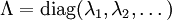$\Lambda = \mathrm{diag} (\lambda_1, \lambda_2, \dots)$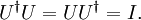$U^\dagger U = U U^\dagger= I.$

The entries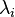$\lambda_i$ of the diagonal matrix$\Lambda$ are the eigenvalues of$A$, and the columns of$U$ are the eigenvectors of$A$. The matching eigenvalues in$\Lambda$ must be ordered as the eigenvectors are ordered as columns of$U$.